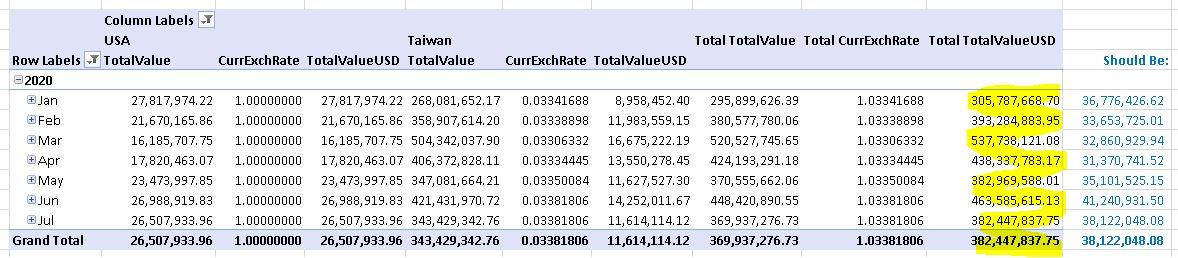Hello all,

I have a fact table containing daily inventory transactions, so I have to add the cumulative records to get the values for a given date.  This is complicated by having transactions from various global divisions with monetary values in the native currencies.  I have USD equivalents of all monetary values by multiplying the cumulative native values by the respective currency rate on the given date.  This is the syntax for the TotalValueUSD calculated measure:

`sum(PeriodsToDate([Calendar].[Yr Mnth Day].[(All)]),[Measures].[TransTotalValue]) * [Measures].[CurrExchRate].[All]`

Everything displays perfectly in a pivot table, except for the row totals.  It appears to be summing the various exchange rates and then multiplying that by the sum of the total transaction value, which totally distorts the result.  Below is an example with the U.S. and Taiwan divisions selected:Would someone tell me how the proper formula/syntax for the TotalValueUSD measure should be so that the proper value appears in the row total?  Thanks.

•JaeW_atOnyx

BBussey you've stumbled on the joy's / agonies of MDX!

You need to alter the SCOPE of your calculated measure to calculate at the 'row level'

Here's a blog post written by the master of SSAS multidimensional Chris Webb.

https://blog.crossjoin.co.uk/2013/05/29/aggregating-the-result-of-an-mdx-calculation-using-scoped-assignments/

Once you've figured out the scoping, just apply it to the scripting block of the SSAS Cubes in Jet Enterprise.

Jae@onyxReporting.com

•JaeW_atOnyx

Note, as Chris says, for best performance, create your Measure as a DERIVED MEASURE (not a CALCULATED MEASURE) and set the calculation to NULL.

Then use your SCOPE statement to define the Derived Measure.  Make sure to use Attribute Hierarchies (in your case, the key level) not User Defined Hierarchies in your SCOPE statement.

This will be a lot of sweat blood and tears if it's your first time, but after you define the Derived Measure, you can "Reapply Script Commands" between implementations instead of re-deploying the cube.

•BBussey

I've actually got the calculated measures working with this syntax:

`Iif(    IsLeaf([Company].CurrentMember),    ([Measures].[IvtyValue] * [Measures].[CurrExchRate]),    Sum    (        CrossJoin        (            [Calendar].[Yr Mnth Day].CurrentMember,            [Company].[Company].[Company].Members        ),         ([Measures].[IvtyValue] * [Measures].[CurrExchRate])    )) `

The only part that isn't working is when a company is filtered out, as the totals still represent as if all companies are selected.  This isn't major for us, as all companies will be chosen in this cube 99.9% of the time.  I definitely will play with this Chris Webb logic though to see if I can get the total column to present the proper totals when all companies are not selected.  Thanks.

•Malcolm Johnson

Hi

My solution was to calculate the additional currency in the data stage up the fact table, it then just became a fixed value set at the date of posting

•JaeW_atOnyx

Malcolm Johnson sometimes the simple solutions are the best :P

That works well if the company uses the exchange rate from the time of posting AND you only need a few exchange rates.